## Water Images

#### Water Images

Direction: In each of the following questions you are given a figure followed by four alternatives (a), (b), (c) and (d). Choose the alternatives which is closely resembles the water image of the given combination.

1. Choose the alternatives which is closely resembles the water image of the given combination.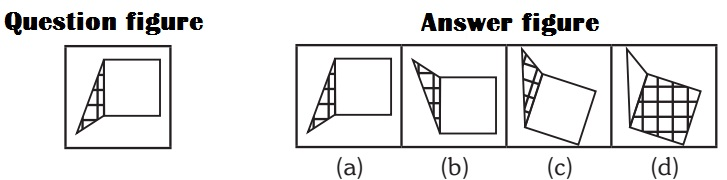1. (c)

##### Correct Option: C

(c)

1. Choose the correct water-image of the figure from responses given below: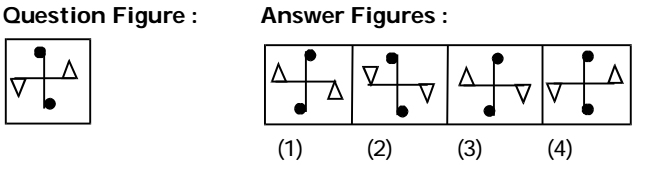1. On the basis of above given question , we know that
Water image is the reflection of an object into the water . It appears by inverting an object vertically i.e. upside down. The water image always be opposite the original image across the given line. It will become opposite to the given line .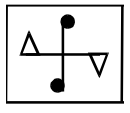##### Correct Option: C

On the basis of above given question , we know that
Water image is the reflection of an object into the water . It appears by inverting an object vertically i.e. upside down. The water image always be opposite the original image across the given line. It will become opposite to the given line .
Hence , option C is required answer .1. Choose the right water-image of the question figure from the given answer figures.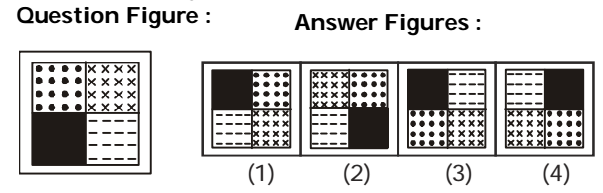1. As we know that the water image always be opposite the original image across the given line. It will become opposite to the given line .As shown in given below .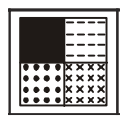##### Correct Option: C

As we know that the water image always be opposite the original image across the given line. It will become opposite to the given line .As shown in given below .
Hence , option C is required answer .1. Which one of the following is water image of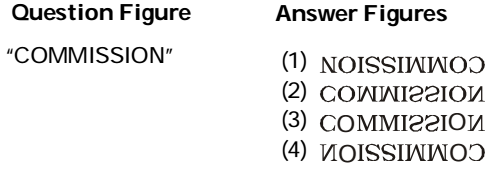1. As per the given above question figure ,
Figure ( 2 ) of the answer figures is exactly the water image of the given original image . Thus , Given image will become opposite in water across the given line.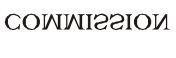##### Correct Option: B

As per the given above question figure ,
Figure ( 2 ) of the answer figures is exactly the water image of the given original image . Thus , Given image will become opposite in water across the given line.So , option B is correct figure .